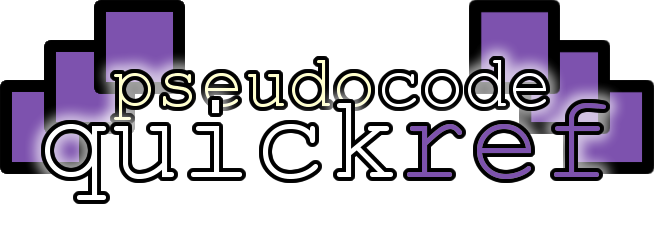Show:

This resource is designed as a quick reference or revision guide. It has not been endorsed by any exam boards. If you spot any mistakes, please let me know and I'll fix them asap.

Repetition / Iteration

Computers are very good at repeating similar tasks very quickly. Writing code that contains loops means your program can repeat certain lines of code without having to write them out over and over again.

There are two types of loop:

• Definite iteration:
When you know exactly how many times a loop will repeat (e.g. repeat exactly 10 times)
• Indefinite iteration
When you don't know exactly how many times a loop will repeat (e.g. repeat until the user presses cancel)

Top tips:

• While loops and repeat until loops both let you repeat code in slightly different ways. You can often choose to use either as long as you understand the differences.
Repeat until loops always run at least once. While loops might never run.
• For loops are great for when you need to iterate through each value in an array.
Iterating means repeating for each value. This can be counting from a minimum to maximum number or going through each value in an array / list.
While loop

A while loop will keep repeating whilst a condition is met (it will stop repeating when the condition is no longer met).

This is known as a pre-conditioned loop because the program checks if the condition has been met before the code in the loop runs. This means that the minimum number of times the loop will run could be 0.

It is also an example of indefinite iteration because the loop could carry on forever unless the condition is met.

This example program keeps asking the user "Are we nearly there yet" until they say "yes"

Repeat Until loop

A repeat until loop will keep repeating until a condition is met.

This is known as a post-conditioned loop because the program checks if the condition has been met after the code in the loop runs. This means that the loop will always run at least once.

It is also an example of indefinite iteration because the loop could carry on forever if the condition is never met.

This example program keeps asking the user "Are we nearly there yet?" until they say "yes"

Repeat ... times

Repeating ... number of times is known as a count controlled loop because you specify exactly how many times the loop will repeat.

Repeat ... times is an example of definite iteration because the number of times the loop repeats is clearly defined.

This example program will repeat the word "Why?" 10 times

For loop

A for loop is a count-controlled loop which means it your code says exactly how many times the loop should repeat.

A for loop will use a variable to count between the minimum and maximum value. This variable is called the index, which is why for loops often use the variable i.

A for loop is an example of definite iteration because the number of times the loop repeats is clearly defined.

This example will count from 10 to 20

For loop (with step)

for loop is a count-controlled loop which means it your code says exactly how many times the loop should repeat.

A for loop will use a variable to count between the minimum and maximum value. This variable is called the index, which is why for loops often use the variable i.

Rather than increasing the index by 1 each time, you can step up by any amount until you reach the maximum.

This example will count down from 10 to 1 then say "Blast off!"

For Each loop

A for each loop means the code in the loop will run once for each item in an array.

This means it is a count-controlled loop because the number of times the loop will run is not dependent on a condition being met: you know how many times it will repeat in advance.

A for each loop is an example of definite iteration because the number of times the loop repeats is clearly defined.

This example will display the lyrics for "The wheels on the ... go round and round"  for an array containing the strings "bus", "car" and "tram"